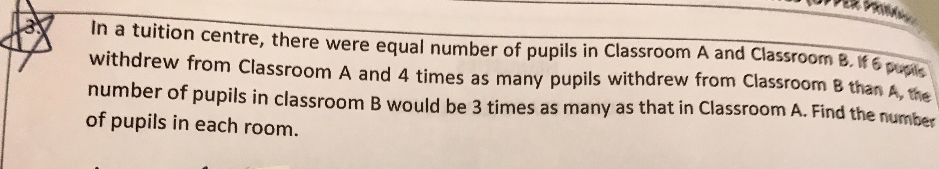# QuestionHi parents, I need help for this questions as we couldn’t solve it, many many thanks!

Source: River Valley Primary

3 Answers

# Answer

The question has an error. It should read as follows : “In a tuition centre, there were equal number of pupils in Classroom A and Classroom B. If 6 pupils withdrew from Classroom A and 4 times as many pupils withdrew from Classroom B than A, the number of pupils in Classroom A would be 3 times as many as that in Classroom B. Find the number of pupils in each room.”

3 – 1 = 2
6 x 4 – 6 = 18
18/2 = 9
9 + 6 x 4 = 33
or
9 x 3 + 6 = 33

Ans : 33 pupils.

0 Replies 0 Likes

Dear vfong1,

There are 2 points in this questions that are not phased correctly.

(1) and 4 times as many pupils withdrew from Classroom B than A. (This phrasing is discouraged to be used many years back already)

Should be 4 times as many pupils withdrew from Classroom B as A.

If not, ‘than’ indicates more by 4 times –> altogether 5 times as many as.

So question comparison requirement is unclear.

(2) number of pupils in Classroom B would be 3 times as many as that in Classroom A.

After more pupils withdrew from Classroom B than A, there should be fewer pupils remained than in A, not more pupils.

0 Replies 0 Likes

This is a Before After question.

 Before Transfer After Class A 1u – 6 1u – 6 Class B 1u – 24 1u – 24

(1u – 6) / 3 =  (1u – 24) / 1

1u – 6 = 3u – 72

72 – 6 = 3u – 1u

2u = 66

1u = 66 ÷ 2 = 33 pupils

This is Table method.

If you are interested to know more, you may search ‘Table Method’ in this site.

0 Replies 1 Like